# 针对毫米波蜂窝系统的信道估计和混合预编码:Channel Estimation and Hybrid Precoding for Millimeter Wave Cellular Systems

《Channel Estimation and Hybrid Precoding for Millimeter Wave Cellular Systems》

## 问题背景

由于在毫米波段大量可用的频率资源，使得毫米波蜂窝系统支持Gbit/s的数据速率。为了实现足够的链路裕度，毫米波系统将在发送端和接收端采用带有大规模天线阵列的定向波束赋形技术。为了减小容量混合处理信号带来的高成本开销和功耗，mmWave precoding将被分为模拟域和数字域。大量天线和模拟波束赋形的存在要求提出特定于mmwave的信道估计和预编码算法。本文利用毫米波信道的低散射性质提出了一种估计毫米波信道参数的自适应算法。使用估计的信道，本文又提出了一种新的混合模拟/数字预编码算法，它能够克服模拟波束赋形的硬件限制并且接近数字解决方案的性能。

## 文章贡献

• 本文利用了毫米波信道的稀疏特性，利用自适应压缩感知(CS) 领域的工具，设计了有效的信道估计算法。
• 为训练预编码器设计了一种创新的多分辨率码本，它依赖于联合模拟/数字处理来保证不同波束宽度的波束向量，对于所提出的自适应信道估计算法也是至关重要的操作。
• 所提出的信道估计算法能够以少的迭代数目，高的成功概率估计信道参数。相较于以前的工作（受限于单波束训练和传输），该算法还能估计多径信道中的信道参数。
• 在单径信道中，推导了信道估计参数错误概率的上界，找到了总训练功率和它在算法自适应阶段的分配的充分条件。
• 针对毫米波信道提出了一种新的混合模拟/数字预编码算法，该算法的设计仅取决用于直逼信道主奇异值向量的量化波束赋形方向
• 假设BS和MS端采用混合预编码算法的情况下，对所提出的估计算法进行了性能分析。

## 系统模型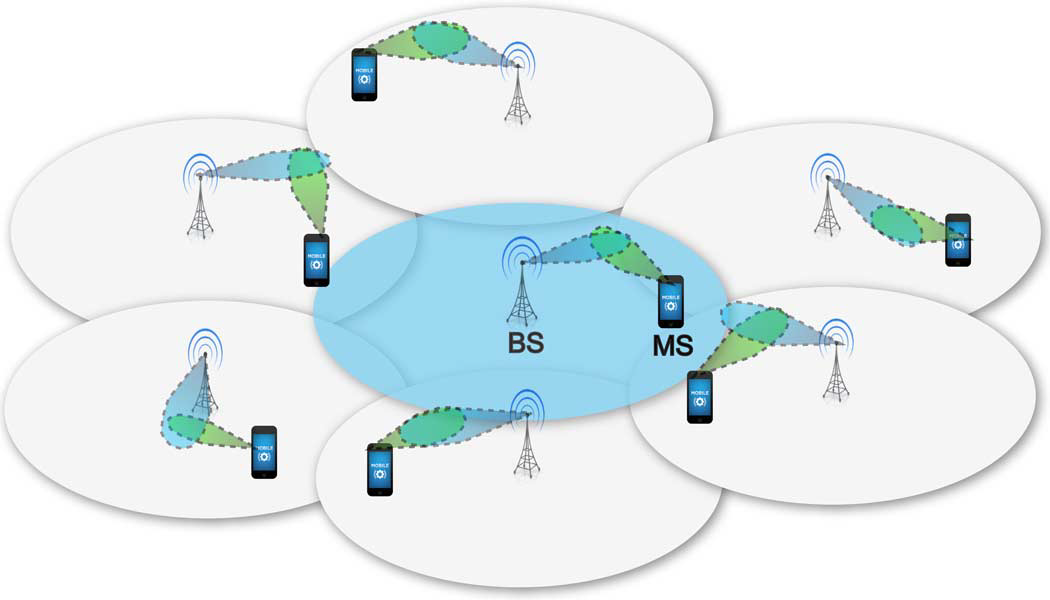考虑如上图所示的毫米波蜂窝系统，基站端和移动用户端具体的配置情况如下框图所示：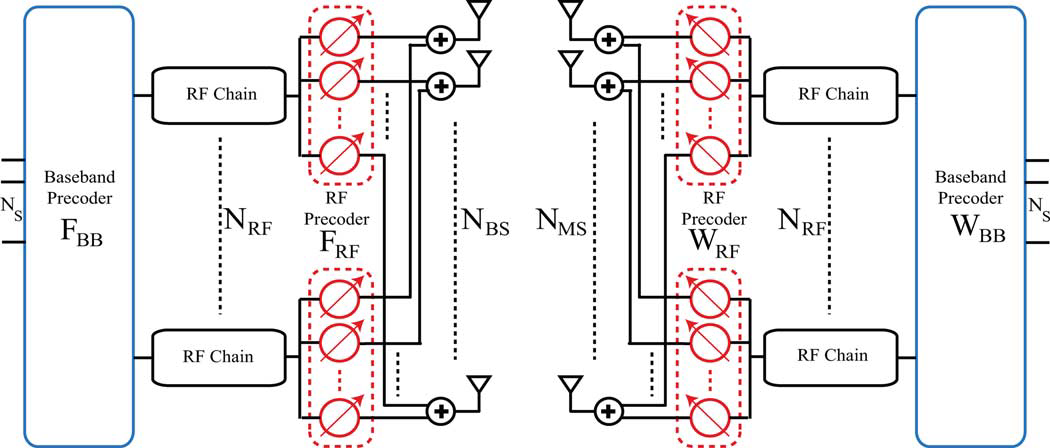在这样一个模型下，信道$\mathbf{H}$可以描述为：
$\mathbf{H}=\sqrt{\frac{N_{\mathrm{BS}} N_{\mathrm{MS}}}{\rho}} \sum_{\ell=1}^{L} \alpha_{\ell} \mathbf{a}_{\mathrm{MS}}\left(\theta_{\ell}\right) \mathbf{a}_{\mathrm{BS}}^{H}\left(\phi_{\ell}\right)$
其中$\rho$代表了从BS到MS的平均路径损耗，$\alpha_{\ell}$$\ell^{t h}$路径的复增益，路径振幅服从瑞丽分布，即$\alpha_{\ell} \sim \mathcal{N}\left(0, \overline{P}_{R}\right)$$\ell=1,2, \ldots, L$$\overline{P}_{R}$是平均功率增益。$\phi_{\ell} \in[0,2 \pi]$$\theta_{\ell} \in[0,2 \pi]$分别是$\ell^{\text { th }}$径BS端离开和MS端到达的方位角（AoDs/AoAs）。文中假设使用的是均匀直线阵，即：$\mathbf{a}_{\mathrm{BS}}\left(\phi_{\ell}\right)=\frac{1}{\sqrt{N_{\mathrm{BS}}}}\left[1, e^{j(2 \pi / \lambda) d \sin \left(\phi_{t}\right)}, \ldots, e^{j\left(N_{\mathrm{BS}}-1\right)(2 \pi / \lambda) d \sin \left(\phi_{t}\right)}\right]^{T}$
$\mathbf{H}$可以表示为如下的一个等价形式：$\mathbf{H}=\mathbf{A}_{\mathrm{MS}} \operatorname{diag}(\boldsymbol{\alpha}) \mathbf{A}_{\mathrm{BS}}^{H}$
其中：$\boldsymbol{\alpha}=\sqrt{N_{\mathrm{BS}} N_{\mathrm{MS}} / \rho}\left[\alpha_{1}, \alpha_{2}, \ldots, \alpha_{L}\right]^{T}$$\mathbf{A}_{\mathrm{BS}}=\left[\mathbf{a}_{\mathrm{BS}}\left(\phi_{1}\right), \mathbf{a}_{\mathrm{BS}}\left(\phi_{2}\right), \ldots, \mathbf{a}_{\mathrm{BS}}\left(\phi_{L}\right)\right]$$\mathbf{A}_{\mathrm{MS}}=\left[\mathbf{a}_{\mathrm{MS}}\left(\theta_{1}\right), \mathbf{a}_{\mathrm{MS}}\left(\theta_{2}\right), \ldots, \mathbf{a}_{\mathrm{MS}}\left(\theta_{L}\right)\right]$

## 毫米波信道估计问题的公式化

上一节对毫米波信道做了一个简要的介绍，后面的章节将主要针对信道估计问题的公式化表达，同时采用基于自适应CS的算法去解决这一问题。对于前面提到的这样一个毫米波信道的几何模型，信道估计相当于估计$L$条信道路径的不同参数，即：AoAs、AoDs、$\alpha_{\ell}$归根结底，就是如何以一个较小的训练开销获得较为精确的信道参数。本文通过利用毫米波信道的低散射特性并将毫米波信道估计问题表示为一个稀疏问题。自适应CS激发了一些关于如何设计训练precoders和combiner的想法，也就是接下来要介绍的针对训练波束向量的分层多分辨率码本。

### A.毫米波信道估计问题的稀疏表达

在BS端使用一个波束向量$\mathbf{f}_{p}$，在MS端使用一个测量向量$\mathbf{w}_{q}$合并接收到的信号，因此得到的信号可以表示为：$y_{q, p}=\mathbf{w}_{q}^{H} \mathbf{H} \mathbf{f}_{p} s_{p}+\mathbf{w}_{q}^{H} \mathbf{n}_{q, p}$  $\mathcal{s}_{p}$是在波束向量$\mathbf{f}_{p}$上的发送信号，满足$\mathbb{E}\left[s_{p} s_{p}^{H}\right]=P$$P$是训练阶段被用于每次传输的平均个功率。现在在进一步的推广，假设在MS端向量$\mathbf{w}_{q}$使用了$M_{\mathrm{MS}}$个这样的测量向量，即：$q=1,2, \dots, M_{\mathrm{MS}}$，用于连续瞬间检测通过波束成形向量$\mathbf{f}_{p}$传输的信号，在连续的$M_{\mathrm{BS}}$个时间段里使用$M_{\mathrm{BS}}$个这样的波束成形向量$\mathbf{f}_{p}$$p=1, \dots, M_{\mathrm{BS}}$。整个过程就可以表示为：$\mathbf{Y}=\mathbf{W}^{H} \mathbf{H F S}+\mathbf{Q}$其中：$\mathbf{F}=\left[\mathbf{f}_{1}, \mathbf{f}_{2}, \ldots, \mathbf{f}_{M_{\mathrm{BS}}}\right]$是被用在BS端大小为$N_{\mathrm{BS}} \times M_{\mathrm{BS}}$波束成形向量，$\mathbf{Q}$是把$M_{\mathrm{BS}}$个噪声向量串联在一起的噪声矩阵，$\mathbf{S}$是携带$M_{\mathrm{BS}}$个传输符号的对角阵。在训练阶段，假定所有的传输符号都是相同的，也就是$\mathbf{S}=\sqrt{P} \mathbf{I}_{M_{\mathrm{BS}}}$，因此可以进一步简化为：$\mathbf{Y}=\sqrt{P} \mathbf{W}^{H} \mathbf{H F}+\mathbf{Q}$$\mathbf{Y}$向量化，然后做一系列的数学推导可以得到最终的如下形式$\mathbf{y}_{v}=\sqrt{P}\left(\mathbf{F}^{T} \otimes \mathbf{W}^{H}\right)\left(\mathbf{A}_{\mathrm{BS}}^{*} \circ \mathbf{A}_{\mathrm{MS}}\right) \boldsymbol{\alpha}+\mathbf{n}_{\mathrm{Q}}$(多说一句：‘’$\circ$‘’是Khatri-Rao 积，$\mathbf{A}_{\mathrm{BS}}^{*} \circ \mathbf{A}_{\mathrm{MS}}$是大小为$N_{\mathrm{BS}} N_{\mathrm{MS}} \times L$的矩阵，它的每一列由$\mathbf{a}_{\mathrm{BS}}^{*}\left(\phi_{\ell}\right) \otimes \mathbf{a}_{\mathrm{MS}}\left(\theta_{\ell}\right)$$\ell=1,2, \ldots, L$）组成，也就是说每一列与第$\ell$条径的AoA/AoD相关)
假设AoAs和AoDs取自均匀网格中的$N$点，即：$\phi_{\ell}, \theta_{\ell} \in\{0,2 \pi / N, \ldots, 2 \pi(N-1) / N\}, \ell=1,2, \ldots, L$注意：AoDs/AoAs实际上是连续的，一些基于网格外的算法可以减少这种量化误差，这里只考虑量化的AoDs/AoAs的情况）忽略了网格的量化误差，$\mathbf{y}_{v}$可以表示为：
$\mathbf{y}_{v}=\sqrt{P}\left(\mathbf{F}^{T} \otimes \mathbf{W}^{H}\right) \mathbf{A}_{\mathrm{D}} \mathbf{z}+\mathbf{n}_{\mathrm{Q}}$特别解释一下：$\mathbf{A}_{\mathrm{D}}$是一个$N_{\mathrm{BS}} N_{\mathrm{MS}} \times N^{2}$的字典矩阵，由上面所说的均匀网格上$N$个点构成。$\mathbf{z}$是一个$N^{2} \times 1$的向量，它携带了关于量化方向上的路径增益。（更明确的说：其实$\mathbf{z}$$L$个非0元素就代表了信道的主要路径且$L \ll N^{2}$，得到了它就得到了路径增益）。
到现在，这个稀疏问题就很明确了，CS的工具就可以用来设计信道估计算法来决定量化的AoAs/AoDs。定义感知矩阵$\boldsymbol{\Psi}=\left(\mathbf{F}^{T} \otimes \mathbf{W}^{H}\right) \mathbf{A}_{\mathrm{D}}$，CS算法的目标就是有效地设计感知矩阵保证能以较高的概率、较少的测量次数恢复向量$\mathbf{z}$的非0元素。为了简化，进一步使用克罗内克积的性质，将该问题写为：\begin{aligned} \mathbf{y}_{v} &=\sqrt{P}\left(\mathbf{F}^{T} \mathbf{A}_{\mathrm{BS}, \mathrm{D}}^{*} \otimes \mathbf{W}^{H} \mathbf{A}_{\mathrm{MS}, \mathrm{D}}\right) \mathbf{z}+\mathbf{n}_{\mathrm{Q}} \\ &=\sqrt{P} \mathbf{F}^{T} \mathbf{A}_{\mathrm{BS}, \mathrm{D}}^{*} \mathbf{Z}_{\mathrm{BS}} \otimes \mathbf{W}^{H} \mathbf{A}_{\mathrm{MS}, \mathrm{D}} \mathbf{z}_{\mathrm{MS}}+\mathbf{n}_{\mathrm{Q}} \end{aligned}其中，$\mathbf{z}_{\mathrm{BS}}$$\mathbf{z}_{\mathrm{MS}}$是两个$N \times 1$的稀疏向量，它们非0元素的位置与AoDs和AoAs相关。$\mathbf{A}_{\mathrm{BS}, \mathrm{D}}$$\mathbf{A}_{\mathrm{MS}, \mathrm{D}}$$N_{\mathrm{BS}} \times N$$N_{\mathrm{MS}} \times N$的字典矩阵。

### B.自适应CS解决方法

训练过程被分为很多阶段。每个阶段使用的训练预编码和测量矩阵不是预先确定的，而是依赖于早期阶段的输出（自适应的思想）。基于二分法的概念，本文自适应地设计了训练波束成形矢量，经历多次分割后能够在较后的阶段得到一个较小的划分直到在要求的分辨率内找到AoAs/AoDs的非0元素。

## 基于多分辨率分层码本的混合预编码

以前仅模拟的多分辨率码本在mmWave中受到一些实际的限制

• 量化移相器的存在使得设计非重叠的波束模式变得很困难，在大规模天线的引入后会要求在一个很大的搜索空间内进行搜索。
• 很难应用到非ULAs，由于缺少对他们波束模式的直观认识。
下面主要介绍在BS端的训练预编码码本$\mathcal{F}$，同样的方法是可以用到MS端构造训练码本$\mathcal{W}$

### A.码本结构

简单说来，分层码本有$\mathbf{S}$层，$\mathcal{F}_{s}, s=1,2, \ldots, \mathrm{S}$。每一层都包含了具有一定波束宽度的波束向量，它们被用自适应毫米波信道估计算法相关的训练阶段。文中展示了一个$N=256$$K=2$分层码本的例子。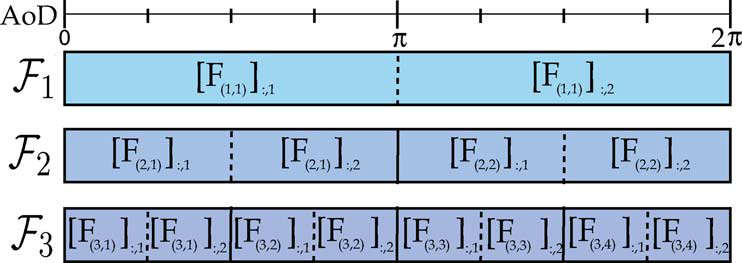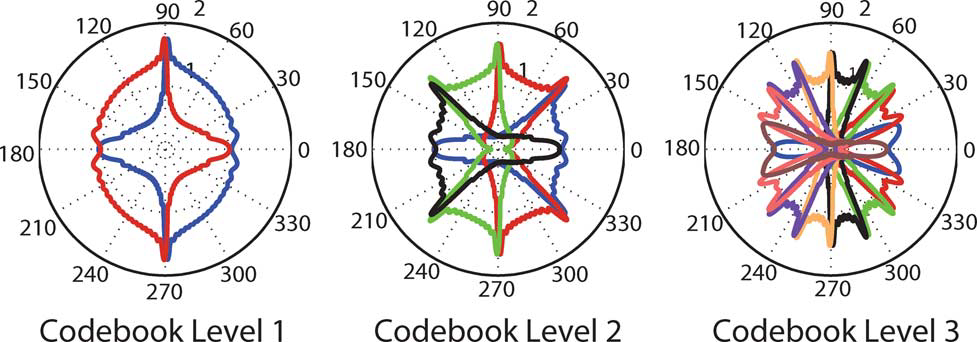### B.码本波束向量的设计

在每一层码本$\mathbf{s}$，每个子集$k$中，波束向量$\left[\mathbf{F}_{(s, k)}\right]_{ :, m}, m=1,2, \ldots, K$被设计成如下的形式：
$\left[\mathbf{F}_{(s, k)}\right]_{ ;, m}^{H} \mathbf{a}_{\mathrm{BS}}\left(\overline{\phi}_{u}\right)=\left\{\begin{array}{ll}{C_{s}} & {\text { if } u \in \mathcal{I}_{(s, k, m)}} \\ {0} & {\text { if } u \notin \mathcal{I}_{(s, k, m)}}\end{array}\right.$其中$\mathcal{I}_{(k, s, m)}=\left\{\frac{N}{K^{s}}(K(k-1)+m-1)+1, \ldots, \frac{N}{K^{s}}(K(k-1)+m)\right\}$定义了与波束向量$\left[\mathbf{F}_{(s, k)}\right]_{ :, m}$相关的AoDs子范围，$C_{s}$是归一化约束，满足$\left\|\mathbf{F}_{(s, k)}\right\|_{F}=K$
将上述的形式进一步表示为：
$\mathbf{A}_{\mathrm{BS}, \mathrm{D}}^{H} \mathbf{F}_{(s, k)}=C_{s} \mathbf{G}_{(s, k)}$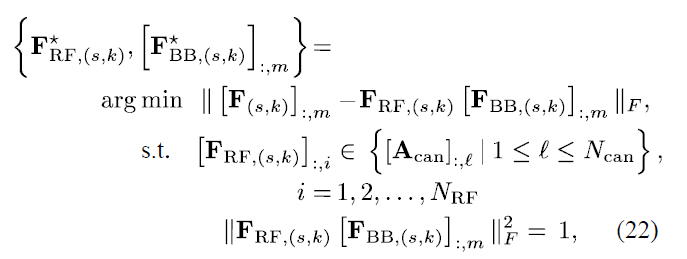## 针对毫米波信道的自适应估计算法

### 单径

单径时，对于前面提到的$\mathbf{z}$来说就只剩下一个非0的元素，确定0元素的位置就等于完成了信道估计。为了以一个较低的训练开销完成这个过程，本文针对单径的信道估计提出了算法1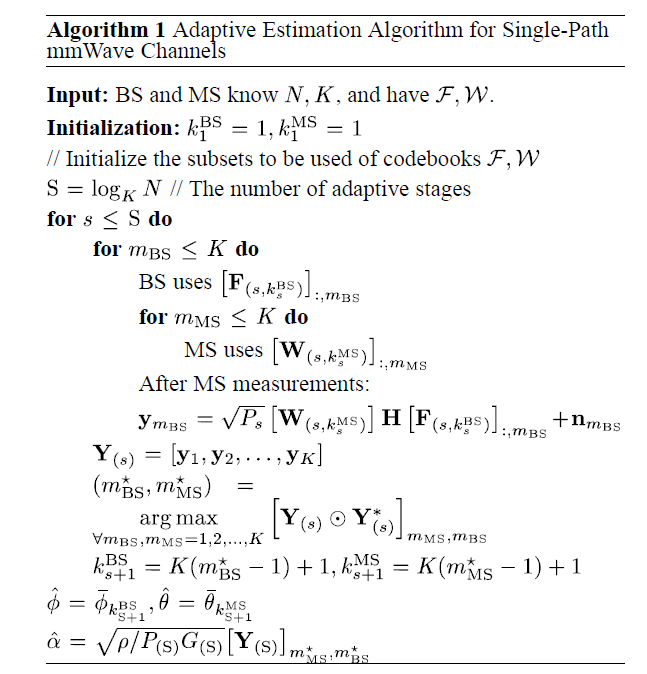后续的内容是对单径的性能进行分析，较为复杂，感兴趣的读者可以参照原文。

### 多径

本节提出了一种改进的匹配追踪算法来估计AoAs、AoDs和相关径的路径增益。下面做一个简要的介绍，自适应算法在多径的情况下是在BS端和MS端使用$K L_{d}$而不是$K$$L_{d}$是需要求解的主导路径的数量）个预编码和测量向量。在每一个阶段中，$K L_{d}$个分区的$L_{d}$个被选择用于下一阶段更精细的划分。下面直接来看算法：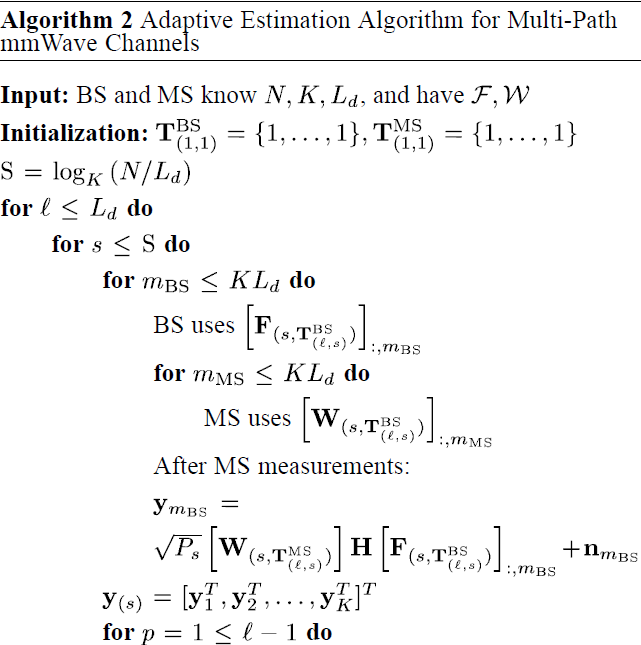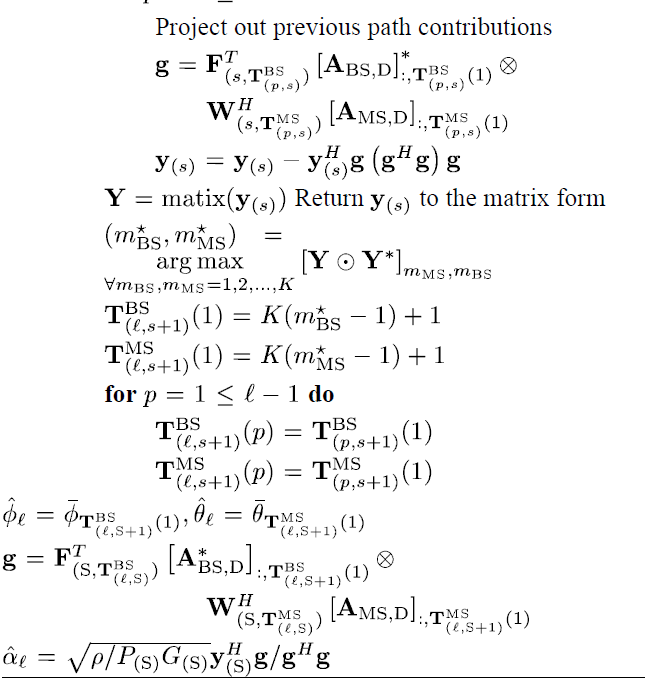## 混合预编码设计

03-12228

#### 学习笔记：OMP算法（Spatially Sparse Precoding in Millimeter wave MIMO Systems）

03-227万+

#### 男生更看重女生的身材脸蛋，还是思想？07-141459

#### 部分连接时MIMO的混合预编码:Hybrid Precoding for mmWave Massive MIMO Systems With Partially-Connected Structure

03-112040

#### 基于压缩感知的Massive mmWave Massive MIMO信道估计学习总结

06-05387

#### SISO/MIMO信道估计（channel estimation）原理详细图解

02-152万+

#### 强烈推荐 10 款珍藏的 Chrome 浏览器插件

04-122246

#### 毫米波蜂窝系统的信道估计及混合预编码

03-082万+

#### 85后蒋凡：28岁实现财务自由、34岁成为阿里万亿电商帝国双掌门，他的人生底层逻辑是什么？...

09-17983

#### 毫米波信道的稀疏性分析

12-04121

#### Geometric Mean Decomposition Based Hybrid precoding for mmWave mMIMO

04-184万+

#### 深度通信网络专栏： ComNet for OFDM接收机——深度学习与传统理论的结合

03-185万+

#### 带了6个月的徒弟当了面试官，而身为高级工程师的我天天修Bug......

02-216万+

#### Python可以这样学（第一季：Python内功修炼）

02-23©️2020 CSDN 皮肤主题: 大白 设计师: CSDN官方博客点击重新获取扫码支付1.余额是钱包充值的虚拟货币，按照1:1的比例进行支付金额的抵扣。
2.余额无法直接购买下载，可以购买VIP、C币套餐、付费专栏及课程。余额充值